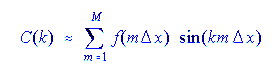## A Comparison of Grid Point and Spectral Models Formulations:

### Spectral Model

1. A field is represented by its value at discrete grid points.

.

f = f(m dx)

Grid points:
fm         fm+1
*             *

1. A field is expressed using a discrete set of coefficients of known functions.In this example, the basis functions are sines.

2. Calculation is done in "real" space (at grid points)
2. Calculation is done in "phase" space (sometimes called "spectral" space) and also in real space

3. Derivatives are by finite differences:3. Derivatives are by summing derivatives of each basis function:4. Increase the resolution by choosing a smaller grid point interval: i.e. smaller dx. This means more grid points at which to make calculations.
4. Increase the resolution by choosing a larger maximum wavenumber k. (Larger k is a smaller wavelength.)